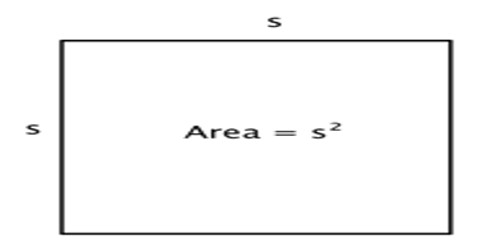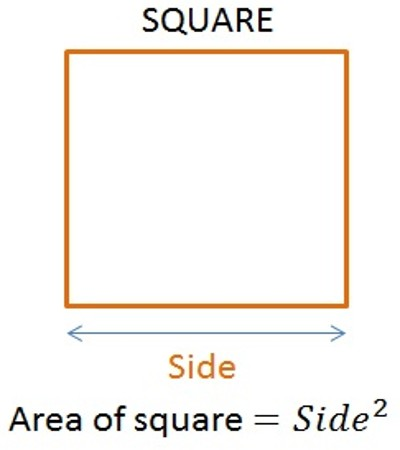Mathematic

# Area of a SquareArea of a Square

A square is a regular polygon with four sides. It has four right angles and parallel sides. To calculate the area of a square, multiply the base by itself, which can be expressed as side × side. If a square has a base of length 8 inches its area will be 8 × 8 = 64 square inches.

Area of a square is given by: A = a2

where a = length of side.In strictly correct mathematical wording the formula above should be spoken as “s raised to the power of 2”, meaning s is multiplied by itself. But we usually say it as “s squared”. This wording actually comes from the square. The length of a line s multiplied by itself, creates the square of side s. Hence “s squared”.

Calculating the Area of a Square

• The area of a square can be found by multiplying the base times itself. This is similar to the area of a rectangle but the base is the same length as the height.
• If a square has a base of length 6 inches its area is 6*6=36 square inches.

Example: Calculate the area of square, where the square has 35cm side’s length.

Solution:

Area of square is defined by a × a.

Area = 35 × 35

Area = 1225 cm.

Information source: